|

# 办公室玄关旺财字画挂什么?画虽小却暗藏乾坤

玄关是办公人员出入的必经场所，也是外界能量进入办公室中的必经之路，这个位置吉凶与否，给予办公环境很大的影响。玄关的装修风格宜简约、大方，对玄关进行装修,应根据办公室本身的结构来决定玄关的风格,最好简洁、大方,如果玄关是一条狭长的独立空间,则可以采用多种装修风格，如果玄关与厅堂相连，没有明显的独立空间，可利用间隔将其分开,并制造独特的风格,也可以与厅堂的装修风格相统一,如果玄关以及包含在厅堂里宜与厅堂的装修风格相统一,与此同时应对玄关进行画龙点睛式的修饰,为厅堂增加亮点。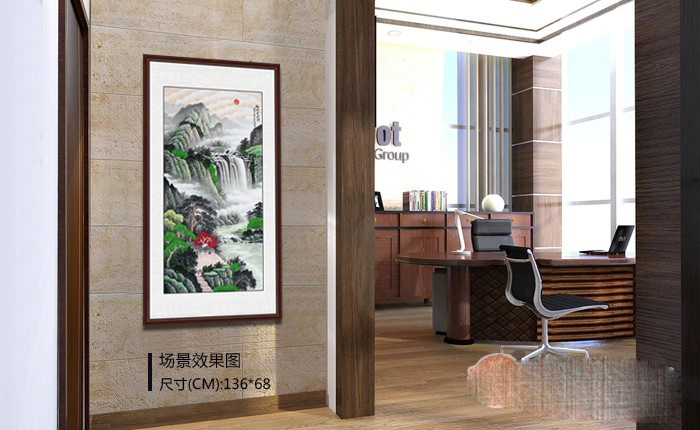玄关给来访者留下的第一印象,少而精的饰品可以起到画龙点睛的作用,一只小花瓶,一束干树枝,可给玄关增添几分灵气,一幅精美的挂画,都能体现出主人的品位与修养,但是注意,由于玄关位居冲要,对宅运大有影响,因此，摆放在此处的饰物要以寓意吉祥之物为佳。古人多摆放狮子,麒麟这些威猛而具有灵性的猛兽在门口镇守,作为住宅的守护神,现代住宅如果摆放狮子或麒麟在屋外,往往会受到诸多限制,退而求其次,则可摆入风水画在玄关内而向大门之处,同样也可收护宅之效，玄关挂什么画风水好?办公室你得这样选!

办公室进门处玄关挂画推荐：

李林宏最新力作四尺竖幅风水挂画《福地安居图》作品来源：易从网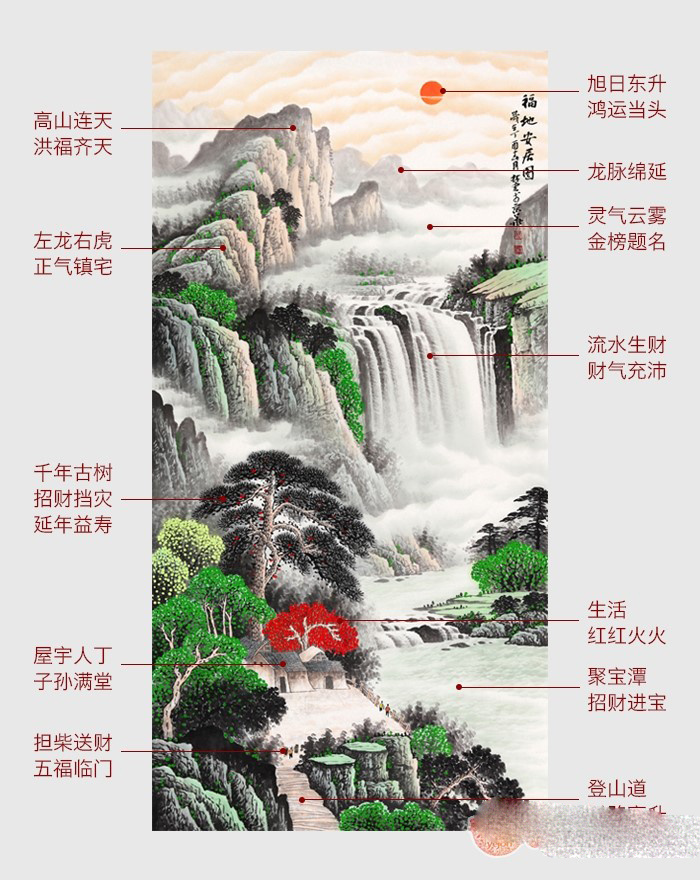山水画是国画中最具品位的了，以自然山水为基本的描绘对象，给我们一种安静祥和的舒适之感，并且山水画和中国的风水更是紧密相连，山主丁水主财也是山水画的吉祥寓意，并且这幅玄关装饰画是以绿色为主色彩，给人一中的按自然的辛勤和生机，使人感到轻松愉快。尤其是其中风水寓意极佳!堪称完美!

旭日东升，鸿运当头事业蒸蒸日上!龙脉绵延，飞龙吐珠官印在握!

坚实靠山，鸿运齐天贵人相助!祥云天降，瑞气千条吉祥相伴!

流水生财，财运滚滚财气充沛!担柴送财，财路进财五福临门!

财路高升，步步登高事业兴旺!屋宇人丁，富贵吉祥子孙满堂!

灵气云雾，人杰地灵金榜题名!树木林立，挡灾招财更具生机!

开门见春，春意盎然，旭日东升，浩然正气，挂在正玄关更是镇宅气势之选。

办公室进门处玄关挂画推荐：

李林宏最新力作旺运山水画《鸿运当头》作品来源：易从网“鸿运当头”这个四个字在中国的字典里本来就是一个富有吉祥寓意的成语，所谓鸿运当头，就是能给大家带来好运，使得个人和家庭甚至单位都可以好运不断，生生不息。在中国人几千年的悠久历史中“红”文化源远流长，红色总能给人们带来希望，当人们的意识在最低谷的时候，看到红色的东西，总能感受到无限的温暖，使人再次充满生机与活力。

开门见红，好运连连，鸿运当头山水画是中国著名的十大风水画之一，玄关素来就是一个藏风纳气的地方，对于办公室中的风水影响颇大，所以一幅好的玄关风水画是尤为重要的，李林宏这幅鸿运当头山水画以朱砂红点缀各个山头，描绘出万山红遍的景象，犹如一个个跳动的红精灵，吉祥如意，红日当空，浩然云雾充斥山涧，祥瑞之兆，山上的松树有着招财迎客挡灾的寓意，步步高升的登山道，这样好风水的山水画挂在玄关绝佳!

办公室进门处玄关挂画推荐：

小品斗方画 李林宏新作山水画《迎客松》作品来源：易从网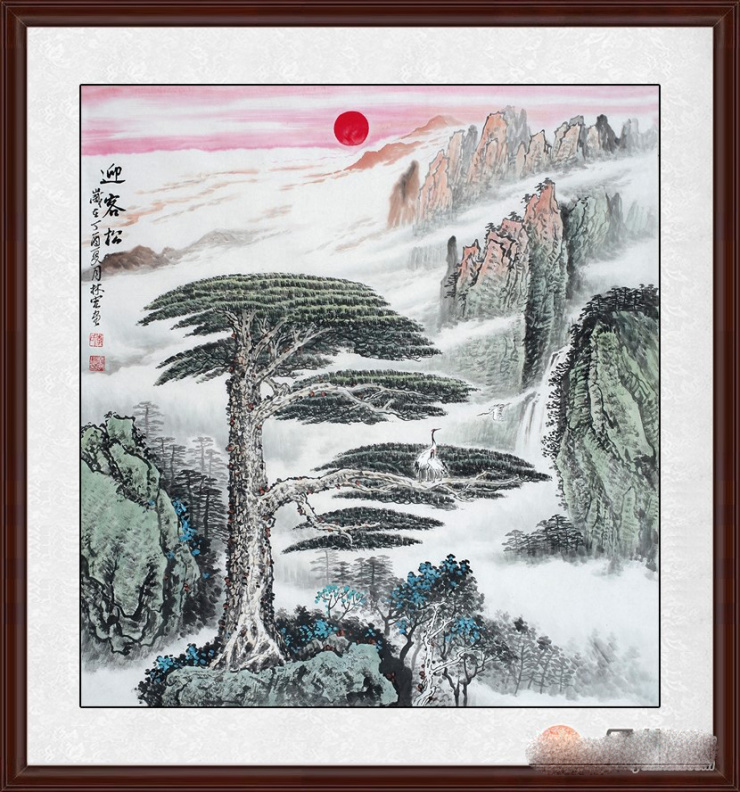奇松傲立玉屏前，阅尽沧桑色更鲜。双臂垂迎天下客，包容四海寿千年。

君子之正气选择，黄山迎客松，桀骜不驯，英姿飒爽，谦恭有礼!

迎客松也是一种欢迎宾客到来之意，所以挂在家中极为合适，谦恭有礼，君子大方之气，这幅画将浩荡的天地之间氤氲之气跃入画卷，灵气，福气，财气将相随融通，丰泽绵绵。迎客松在风水学中，寓意指迎福招财。挂在家中遮灾避难，旺财运。中国人视松为吉祥物,松被视作“百木之长”,称作“木公”、“大夫”.松的特点是凌霜不凋、冬夏常青.苍松劲挺,饱含风霜而生机勃勃!因此,古人视松作长青之树,古代有长生不老松之说,人们赋予其延年益寿、长青不老的吉祥寓意.松也是吉祥的梦兆.松更普遍地是被视作祝颂、祈盼青春永驻、健康长寿的象征物。

据《雀豹古今注》中载，“鹤千年则变成苍，又两千岁则变黑，所谓玄鹤也”。可见古人认为鹤是多么长寿了，因而鹤常被认为鸟中长寿的代表。松在古代人们心目中认为是百木之长，在古籍中亦有载“松柏之有心也，贯四时而不改柯易叶。”所以，松除了是一种长寿的象征外，也常常作为有志有节的代表和象征。松鹤延年则寓延年益寿或为志节清高之意。亦有称“松鹤同春”。此幅作品不仅适合挂在办公室，更是祝寿礼品的首选!

办公室进门处玄关挂画推荐：

玄关挂画一进门 李林宏流水生财聚宝盆《祥瑞聚福图》作品来源：易从网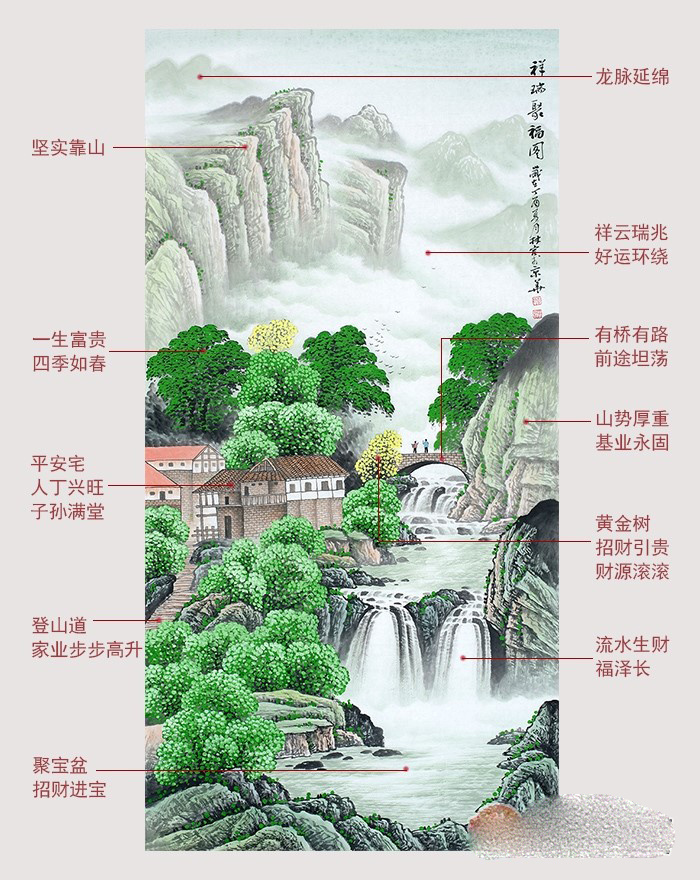都说开门见喜，喜从何来呢?那么春景怡人的景色绝对是长期雾霾中的人们最喜欢的景色，那么李林宏的这幅《祥瑞聚福图》给人最具生机的感觉就是极佳的选择了，满眼绿色一种生命力的象征，小桥流水人家，桃源诗意也就是如此吧，下方的流水汇聚为一潭，有聚宝盆的聚财之意，青山秀水人杰灵，山水常在，杰灵常在，山水画让你的玄关熠熠生辉，财运滚滚!

李林宏老师的这幅《祥瑞聚福图》山水画作为玄关或者走廊挂画极为合适，先抛开其他的，就看其中的青山秀水就是一种浩然正气的存在，玄关挡煞绿意葱葱绝对是最佳的选择，给人一种正大光明的感觉，飞流的瀑布，开门见财运，满眼的春意盎然，无论是自己还是客人绝对都会有耳目一新的感觉!玄关的特殊性，我们不宜选择太大画，作为玄关挂画，一般可选画为竖幅作品，横幅小品等等都是不错的选择。玄关山水画，旺财运，开势运，兴声运!更多适合办公室玄关挂的风水画选择尽在易从网!

好消息!!!2017年【易从网】定制服务升级啦!

1、来图即可定制

假如我们商城没有您喜欢的山水画构图，您只需把高清图片发给客服，我们会安排签约的知名画家为您“一对一”创作，比如以“桃源山水画”而著称的画家石开、以“长城山水画”而著称的画家李林宏、以“青绿山水画”而著称的画家吴大恺等。

2、作品落款定制

若您看上了我们网站的山水画，却因个人爱好或者场合需要，想换个作品名字或者想加添几个字，比如领导升职时，您准备购买李林宏老师的长城山水画《中华之魂》当做礼物，可定制落款成《步步高升》，并加上自己的名字。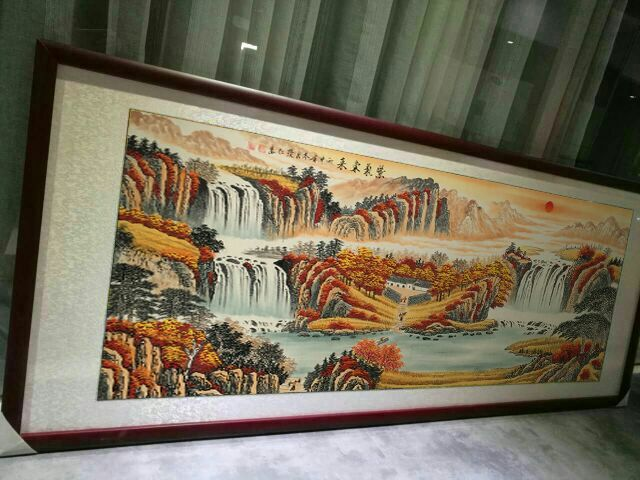——客户李林宏《紫气东来》山水画实拍

3、规格尺寸定制

比如您家客厅比较大，装修时也是专门定制的长沙发，不用担心，我们的画家从斗方小尺到五米巨幅山水画都有，毕竟山水画大的才更气派，您将所需的作品尺寸发给在线客服，便可以为您预约老师定制。

【易从网】，一家致力于传播中国传统书画文化，引领当代书画艺术品运用于装饰、送礼、收藏的专业字画商城。“让艺术走近大众，提升国民艺术水平”是我们的使命和追求。

`声明：本文由入驻焦点开放平台的作者撰写，除焦点官方账号外，观点仅代表作者本人，不代表焦点立场错误信息举报电话： 400-099-0099，邮箱：jubao@vip.sohu.com，或点此进行意见反馈，或点此进行举报投诉。`A B C D E F G H J K L M N P Q R S T W X Y Z
A - B - C - D - E
• A
• 鞍山
• 安庆
• 安阳
• 安顺
• 安康
• 澳门
• B
• 北京
• 保定
• 包头
• 巴彦淖尔
• 本溪
• 蚌埠
• 亳州
• 滨州
• 北海
• 百色
• 巴中
• 毕节
• 保山
• 宝鸡
• 白银
• 巴州
• C
• 承德
• 沧州
• 长治
• 赤峰
• 朝阳
• 长春
• 常州
• 滁州
• 池州
• 长沙
• 常德
• 郴州
• 潮州
• 崇左
• 重庆
• 成都
• 楚雄
• 昌都
• 慈溪
• 常熟
• D
• 大同
• 大连
• 丹东
• 大庆
• 东营
• 德州
• 东莞
• 德阳
• 达州
• 大理
• 德宏
• 定西
• 儋州
• 东平
• E
• 鄂尔多斯
• 鄂州
• 恩施
F - G - H - I - J
• F
• 抚顺
• 阜新
• 阜阳
• 福州
• 抚州
• 佛山
• 防城港
• G
• 赣州
• 广州
• 桂林
• 贵港
• 广元
• 广安
• 贵阳
• 固原
• H
• 邯郸
• 衡水
• 呼和浩特
• 呼伦贝尔
• 葫芦岛
• 哈尔滨
• 黑河
• 淮安
• 杭州
• 湖州
• 合肥
• 淮南
• 淮北
• 黄山
• 菏泽
• 鹤壁
• 黄石
• 黄冈
• 衡阳
• 怀化
• 惠州
• 河源
• 贺州
• 河池
• 海口
• 红河
• 汉中
• 海东
• 怀来
• I
• J
• 晋中
• 锦州
• 吉林
• 鸡西
• 佳木斯
• 嘉兴
• 金华
• 景德镇
• 九江
• 吉安
• 济南
• 济宁
• 焦作
• 荆门
• 荆州
• 江门
• 揭阳
• 金昌
• 酒泉
• 嘉峪关
K - L - M - N - P
• K
• 开封
• 昆明
• 昆山
• L
• 廊坊
• 临汾
• 辽阳
• 连云港
• 丽水
• 六安
• 龙岩
• 莱芜
• 临沂
• 聊城
• 洛阳
• 漯河
• 娄底
• 柳州
• 来宾
• 泸州
• 乐山
• 六盘水
• 丽江
• 临沧
• 拉萨
• 林芝
• 兰州
• 陇南
• M
• 牡丹江
• 马鞍山
• 茂名
• 梅州
• 绵阳
• 眉山
• N
• 南京
• 南通
• 宁波
• 南平
• 宁德
• 南昌
• 南阳
• 南宁
• 内江
• 南充
• P
• 盘锦
• 莆田
• 平顶山
• 濮阳
• 攀枝花
• 普洱
• 平凉
Q - R - S - T - W
• Q
• 秦皇岛
• 齐齐哈尔
• 衢州
• 泉州
• 青岛
• 清远
• 钦州
• 黔南
• 曲靖
• 庆阳
• R
• 日照
• 日喀则
• S
• 石家庄
• 沈阳
• 双鸭山
• 绥化
• 上海
• 苏州
• 宿迁
• 绍兴
• 宿州
• 三明
• 上饶
• 三门峡
• 商丘
• 十堰
• 随州
• 邵阳
• 韶关
• 深圳
• 汕头
• 汕尾
• 三亚
• 三沙
• 遂宁
• 山南
• 商洛
• 石嘴山
• T
• 天津
• 唐山
• 太原
• 通辽
• 铁岭
• 泰州
• 台州
• 铜陵
• 泰安
• 铜仁
• 铜川
• 天水
• 天门
• W
• 乌海
• 乌兰察布
• 无锡
• 温州
• 芜湖
• 潍坊
• 威海
• 武汉
• 梧州
• 渭南
• 武威
• 吴忠
• 乌鲁木齐
X - Y - Z
• X
• 邢台
• 徐州
• 宣城
• 厦门
• 新乡
• 许昌
• 信阳
• 襄阳
• 孝感
• 咸宁
• 湘潭
• 湘西
• 西双版纳
• 西安
• 咸阳
• 西宁
• 仙桃
• 西昌
• Y
• 运城
• 营口
• 盐城
• 扬州
• 鹰潭
• 宜春
• 烟台
• 宜昌
• 岳阳
• 益阳
• 永州
• 阳江
• 云浮
• 玉林
• 宜宾
• 雅安
• 玉溪
• 延安
• 榆林
• 银川
• Z
• 张家口
• 镇江
• 舟山
• 漳州
• 淄博
• 枣庄
• 郑州
• 周口
• 驻马店
• 株洲
• 张家界
• 珠海
• 湛江
• 肇庆
• 中山
• 自贡
• 资阳
• 遵义
• 昭通
• 张掖
• 中卫

1室1厅1厨1卫1阳台

1
2
3
4
5

0
1
2

1

1

0
1
2
3报名成功，资料已提交审核A B C D E F G H J K L M N P Q R S T W X Y Z
A - B - C - D - E
• A
• 鞍山
• 安庆
• 安阳
• 安顺
• 安康
• 澳门
• B
• 北京
• 保定
• 包头
• 巴彦淖尔
• 本溪
• 蚌埠
• 亳州
• 滨州
• 北海
• 百色
• 巴中
• 毕节
• 保山
• 宝鸡
• 白银
• 巴州
• C
• 承德
• 沧州
• 长治
• 赤峰
• 朝阳
• 长春
• 常州
• 滁州
• 池州
• 长沙
• 常德
• 郴州
• 潮州
• 崇左
• 重庆
• 成都
• 楚雄
• 昌都
• 慈溪
• 常熟
• D
• 大同
• 大连
• 丹东
• 大庆
• 东营
• 德州
• 东莞
• 德阳
• 达州
• 大理
• 德宏
• 定西
• 儋州
• 东平
• E
• 鄂尔多斯
• 鄂州
• 恩施
F - G - H - I - J
• F
• 抚顺
• 阜新
• 阜阳
• 福州
• 抚州
• 佛山
• 防城港
• G
• 赣州
• 广州
• 桂林
• 贵港
• 广元
• 广安
• 贵阳
• 固原
• H
• 邯郸
• 衡水
• 呼和浩特
• 呼伦贝尔
• 葫芦岛
• 哈尔滨
• 黑河
• 淮安
• 杭州
• 湖州
• 合肥
• 淮南
• 淮北
• 黄山
• 菏泽
• 鹤壁
• 黄石
• 黄冈
• 衡阳
• 怀化
• 惠州
• 河源
• 贺州
• 河池
• 海口
• 红河
• 汉中
• 海东
• 怀来
• I
• J
• 晋中
• 锦州
• 吉林
• 鸡西
• 佳木斯
• 嘉兴
• 金华
• 景德镇
• 九江
• 吉安
• 济南
• 济宁
• 焦作
• 荆门
• 荆州
• 江门
• 揭阳
• 金昌
• 酒泉
• 嘉峪关
K - L - M - N - P
• K
• 开封
• 昆明
• 昆山
• L
• 廊坊
• 临汾
• 辽阳
• 连云港
• 丽水
• 六安
• 龙岩
• 莱芜
• 临沂
• 聊城
• 洛阳
• 漯河
• 娄底
• 柳州
• 来宾
• 泸州
• 乐山
• 六盘水
• 丽江
• 临沧
• 拉萨
• 林芝
• 兰州
• 陇南
• M
• 牡丹江
• 马鞍山
• 茂名
• 梅州
• 绵阳
• 眉山
• N
• 南京
• 南通
• 宁波
• 南平
• 宁德
• 南昌
• 南阳
• 南宁
• 内江
• 南充
• P
• 盘锦
• 莆田
• 平顶山
• 濮阳
• 攀枝花
• 普洱
• 平凉
Q - R - S - T - W
• Q
• 秦皇岛
• 齐齐哈尔
• 衢州
• 泉州
• 青岛
• 清远
• 钦州
• 黔南
• 曲靖
• 庆阳
• R
• 日照
• 日喀则
• S
• 石家庄
• 沈阳
• 双鸭山
• 绥化
• 上海
• 苏州
• 宿迁
• 绍兴
• 宿州
• 三明
• 上饶
• 三门峡
• 商丘
• 十堰
• 随州
• 邵阳
• 韶关
• 深圳
• 汕头
• 汕尾
• 三亚
• 三沙
• 遂宁
• 山南
• 商洛
• 石嘴山
• T
• 天津
• 唐山
• 太原
• 通辽
• 铁岭
• 泰州
• 台州
• 铜陵
• 泰安
• 铜仁
• 铜川
• 天水
• 天门
• W
• 乌海
• 乌兰察布
• 无锡
• 温州
• 芜湖
• 潍坊
• 威海
• 武汉
• 梧州
• 渭南
• 武威
• 吴忠
• 乌鲁木齐
X - Y - Z
• X
• 邢台
• 徐州
• 宣城
• 厦门
• 新乡
• 许昌
• 信阳
• 襄阳
• 孝感
• 咸宁
• 湘潭
• 湘西
• 西双版纳
• 西安
• 咸阳
• 西宁
• 仙桃
• 西昌
• Y
• 运城
• 营口
• 盐城
• 扬州
• 鹰潭
• 宜春
• 烟台
• 宜昌
• 岳阳
• 益阳
• 永州
• 阳江
• 云浮
• 玉林
• 宜宾
• 雅安
• 玉溪
• 延安
• 榆林
• 银川
• Z
• 张家口
• 镇江
• 舟山
• 漳州
• 淄博
• 枣庄
• 郑州
• 周口
• 驻马店
• 株洲
• 张家界
• 珠海
• 湛江
• 肇庆
• 中山
• 自贡
• 资阳
• 遵义
• 昭通
• 张掖
• 中卫• 手机• 分享
• 设计
免费设计
• 计算器
装修计算器
• 入驻
合作入驻
• 联系
联系我们
• 置顶
返回顶部